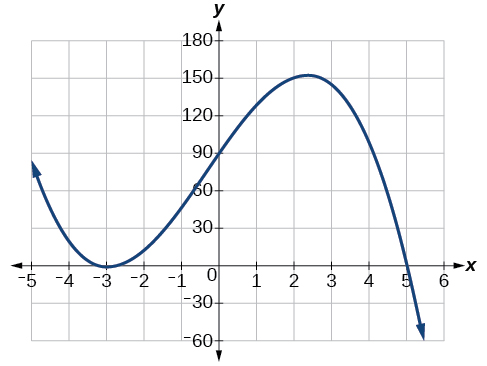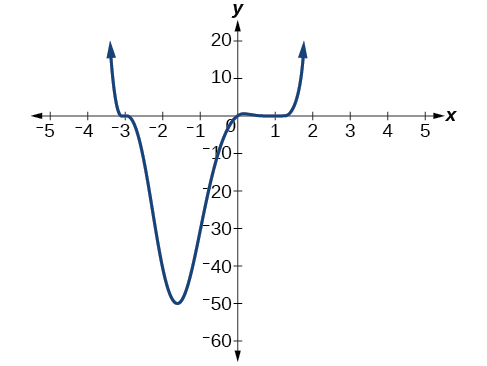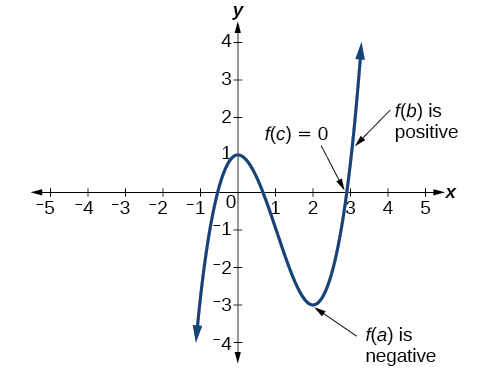# 5.3 Graphs of polynomial functions  (Page 5/13)

 Page 5 / 13

## Sketching the graph of a polynomial function

Sketch a graph of $\text{\hspace{0.17em}}f\left(x\right)=-2{\left(x+3\right)}^{2}\left(x-5\right).$

This graph has two x -intercepts. At $\text{\hspace{0.17em}}x=-3,\text{\hspace{0.17em}}$ the factor is squared, indicating a multiplicity of 2. The graph will bounce at this x -intercept. At $\text{\hspace{0.17em}}x=5,\text{\hspace{0.17em}}$ the function has a multiplicity of one, indicating the graph will cross through the axis at this intercept.

The y -intercept is found by evaluating $\text{\hspace{0.17em}}f\left(0\right).$

$\begin{array}{ccc}\hfill f\left(0\right)& =& -2{\left(0+3\right)}^{2}\left(0-5\right)\hfill \\ & =& -2\cdot 9\cdot \left(-5\right)\hfill \\ & =& 90\hfill \end{array}$

The y -intercept is $\text{\hspace{0.17em}}\left(0,90\right).$

Additionally, we can see the leading term, if this polynomial were multiplied out, would be $\text{\hspace{0.17em}}-2{x}^{3},\text{\hspace{0.17em}}$ so the end behavior is that of a vertically reflected cubic, with the outputs decreasing as the inputs approach infinity, and the outputs increasing as the inputs approach negative infinity. See [link] .

To sketch this, we consider that:

• As $\text{\hspace{0.17em}}x\to -\infty \text{\hspace{0.17em}}$ the function $\text{\hspace{0.17em}}f\left(x\right)\to \infty ,\text{\hspace{0.17em}}$ so we know the graph starts in the second quadrant and is decreasing toward the $\text{\hspace{0.17em}}x\text{-}$ axis.
• Since $\text{\hspace{0.17em}}f\left(-x\right)=-2{\left(-x+3\right)}^{2}\left(-x–5\right)\text{\hspace{0.17em}}$ is not equal to $\text{\hspace{0.17em}}f\left(x\right),\text{\hspace{0.17em}}$ the graph does not display symmetry.
• At $\text{\hspace{0.17em}}\left(-3,0\right),\text{\hspace{0.17em}}$ the graph bounces off of the x -axis, so the function must start increasing.

At $\text{\hspace{0.17em}}\left(0,90\right),\text{\hspace{0.17em}}$ the graph crosses the y -axis at the y -intercept. See [link] .

Somewhere after this point, the graph must turn back down or start decreasing toward the horizontal axis because the graph passes through the next intercept at $\text{\hspace{0.17em}}\left(5,0\right).\text{\hspace{0.17em}}$ See [link] .

As $\text{\hspace{0.17em}}x\to \infty \text{\hspace{0.17em}}$ the function $\text{\hspace{0.17em}}f\left(x\right)\to \mathrm{-\infty },\text{\hspace{0.17em}}$ so we know the graph continues to decrease, and we can stop drawing the graph in the fourth quadrant.

Using technology, we can create the graph for the polynomial function, shown in [link] , and verify that the resulting graph looks like our sketch in [link] .The complete graph of the polynomial function   f ( x ) = − 2 ( x + 3 ) 2 ( x − 5 )

Sketch a graph of $\text{\hspace{0.17em}}f\left(x\right)=\frac{1}{4}x{\left(x-1\right)}^{4}{\left(x+3\right)}^{3}.$## Using the intermediate value theorem

In some situations, we may know two points on a graph but not the zeros. If those two points are on opposite sides of the x -axis, we can confirm that there is a zero between them. Consider a polynomial function $\text{\hspace{0.17em}}f\text{\hspace{0.17em}}$ whose graph is smooth and continuous. The Intermediate Value Theorem    states that for two numbers $\text{\hspace{0.17em}}a\text{\hspace{0.17em}}$ and $\text{\hspace{0.17em}}b\text{\hspace{0.17em}}$ in the domain of $\text{\hspace{0.17em}}f,$ if $\text{\hspace{0.17em}}a and $f\left(a\right)\ne f\left(b\right),$ then the function $\text{\hspace{0.17em}}f\text{\hspace{0.17em}}$ takes on every value between $\text{\hspace{0.17em}}f\left(a\right)\text{\hspace{0.17em}}$ and $\text{\hspace{0.17em}}f\left(b\right).\text{\hspace{0.17em}}$ (While the theorem is intuitive, the proof is actually quite complicated and requires higher mathematics.) We can apply this theorem to a special case that is useful in graphing polynomial functions. If a point on the graph of a continuous function $\text{\hspace{0.17em}}f\text{\hspace{0.17em}}$ at $\text{\hspace{0.17em}}x=a\text{\hspace{0.17em}}$ lies above the $\text{\hspace{0.17em}}x\text{-}$ axis and another point at $\text{\hspace{0.17em}}x=b\text{\hspace{0.17em}}$ lies below the $\text{\hspace{0.17em}}x\text{-}$ axis, there must exist a third point between $\text{\hspace{0.17em}}x=a\text{\hspace{0.17em}}$ and $\text{\hspace{0.17em}}x=b\text{\hspace{0.17em}}$ where the graph crosses the $\text{\hspace{0.17em}}x\text{-}$ axis. Call this point This means that we are assured there is a solution $\text{\hspace{0.17em}}c\text{\hspace{0.17em}}$ where $f\left(c\right)=0.$

In other words, the Intermediate Value Theorem tells us that when a polynomial function changes from a negative value to a positive value, the function must cross the $\text{\hspace{0.17em}}x\text{-}$ axis. [link] shows that there is a zero between $\text{\hspace{0.17em}}a\text{\hspace{0.17em}}$ and $\text{\hspace{0.17em}}b.\text{\hspace{0.17em}}$Using the Intermediate Value Theorem to show there exists a zero.

## Intermediate value theorem

Let $\text{\hspace{0.17em}}f\text{\hspace{0.17em}}$ be a polynomial function. The Intermediate Value Theorem    states that if $\text{\hspace{0.17em}}f\left(a\right)\text{\hspace{0.17em}}$ and $\text{\hspace{0.17em}}f\left(b\right)\text{\hspace{0.17em}}$ have opposite signs, then there exists at least one value $\text{\hspace{0.17em}}c\text{\hspace{0.17em}}$ between $\text{\hspace{0.17em}}a\text{\hspace{0.17em}}$ and $\text{\hspace{0.17em}}b\text{\hspace{0.17em}}$ for which $\text{\hspace{0.17em}}f\left(c\right)=0.$

what are you up to?
nothing up todat yet
Miranda
hi
jai
hello
jai
Miranda Drice
jai
aap konsi country se ho
jai
which language is that
Miranda
I am living in india
jai
good
Miranda
what is the formula for calculating algebraic
I think the formula for calculating algebraic is the statement of the equality of two expression stimulate by a set of addition, multiplication, soustraction, division, raising to a power and extraction of Root. U believe by having those in the equation you will be in measure to calculate it
Miranda
state and prove Cayley hamilton therom
hello
Propessor
hi
Miranda
the Cayley hamilton Theorem state if A is a square matrix and if f(x) is its characterics polynomial then f(x)=0 in another ways evey square matrix is a root of its chatacteristics polynomial.
Miranda
hi
jai
hi Miranda
jai
thanks
Propessor
welcome
jai
What is algebra
algebra is a branch of the mathematics to calculate expressions follow.
Miranda
Miranda Drice would you mind teaching me mathematics? I think you are really good at math. I'm not good at it. In fact I hate it. 😅😅😅
Jeffrey
lolll who told you I'm good at it
Miranda
something seems to wispher me to my ear that u are good at it. lol
Jeffrey
lolllll if you say so
Miranda
but seriously, Im really bad at math. And I hate it. But you see, I downloaded this app two months ago hoping to master it.
Jeffrey
which grade are you in though
Miranda
oh woww I understand
Miranda
Jeffrey
Jeffrey
Miranda
how come you finished in college and you don't like math though
Miranda
gotta practice, holmie
Steve
if you never use it you won't be able to appreciate it
Steve
I don't know why. But Im trying to like it.
Jeffrey
yes steve. you're right
Jeffrey
so you better
Miranda
what is the solution of the given equation?
which equation
Miranda
I dont know. lol
Jeffrey
Miranda
Jeffrey
answer and questions in exercise 11.2 sums
how do u calculate inequality of irrational number?
Alaba
give me an example
Chris
and I will walk you through it
Chris
cos (-z)= cos z .
cos(- z)=cos z
Mustafa
what is a algebra
(x+x)3=?
6x
Obed
what is the identity of 1-cos²5x equal to?
__john __05
Kishu
Hi
Abdel
hi
Ye
hi
Nokwanda
C'est comment
Abdel
Hi
Amanda
hello
SORIE
Hiiii
Chinni
hello
Ranjay
hi
ANSHU
hiiii
Chinni
h r u friends
Chinni
yes
Hassan
so is their any Genius in mathematics here let chat guys and get to know each other's
SORIE
I speak French
Abdel
okay no problem since we gather here and get to know each other
SORIE
hi im stupid at math and just wanna join here
Yaona
lol nahhh none of us here are stupid it's just that we have Fast, Medium, and slow learner bro but we all going to work things out together
SORIE
it's 12
what is the function of sine with respect of cosine , graphically
tangent bruh
Steve
cosx.cos2x.cos4x.cos8x
sinx sin2x is linearly dependent
what is a reciprocal
The reciprocal of a number is 1 divided by a number. eg the reciprocal of 10 is 1/10 which is 0.1
Shemmy
Reciprocal is a pair of numbers that, when multiplied together, equal to 1. Example; the reciprocal of 3 is ⅓, because 3 multiplied by ⅓ is equal to 1
Jeza
each term in a sequence below is five times the previous term what is the eighth term in the sequence
I don't understand how radicals works pls
How look for the general solution of a trig functionByByBy OpenStaxBy Stephen VoronBy Vanessa SoledadBy OpenStaxBy Edward BitonBy Katie MontroseBy Brooke DelaneyBy Prateek AshtikarBy Janet ForresterBy Brenna Fike